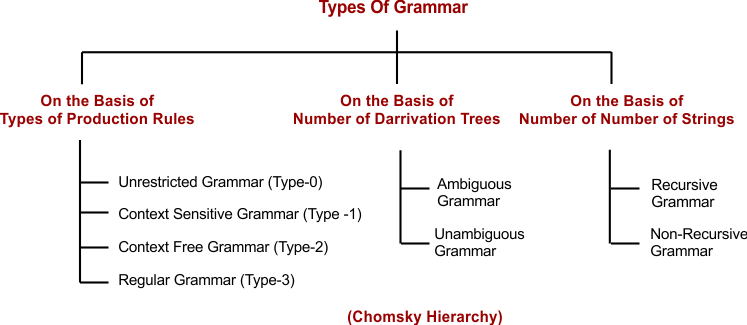Select Page

# Grammar in Automata

Standard way of representing the language is called grammar in Automata. Grammar contains set of production rules which makes the strings of language. The set of all possible strings which can be derived from grammar is known as Language of that grammar.

### Real Life Example of Grammar

Grammar is just like the same as English grammar. If the sentence is correct grammatically then that sentence will be the part of grammar otherwise not. Example

• “I am going to school”. It is a valid example of grammar.
• I going am to school. It is not a valid example of grammar.

## Elements of Grammar

To get better understanding of Grammar, we first understand the elements of grammar. Elements of a grammar depends on depends on types of grammar. The basic elements of grammar are given below.

G = (V, T, P, S) Where V, T, P, S are the elements of Grammar (G).

1. V = Variables (Non-Terminal)

• Finite number of non-empty set Represented by capital symbols. A, B, C
• Not a part of string which makes after production rule.

2. T = Terminal

• Finite set of Alphabets (Σ), Represented by small letters, i.e. a,b,c.
• All variables are replaced with non-terminals through production rules.
• Terminals are part of string which makes after production rule.

3. P = Production Rules

• (Finite set of non-empty rules to makes a string of Language)
• i.e. P = { S → aSb , S → bSa , S → ∈ }

4. S = Start symbol

Start symbol is used to start the production rule represented by S.

Note: Above grammar generates the strings through production rules having equal number of a’s and b’s. As given Below

L = {ab, aabb, aaabbb, …….}

## Equivalent Grammars

Two grammars will be equivalent if and only if they both generate the same languages.

Grammar G1: Consider the Grammar 1 (G1) with following production Rules.

• S → aSb
• S → ∈

Grammar G2: Consider the Grammar 2 (G2) with following production Rules.

• S → aAb / ∈
• A → aAb / ∈

Result: The above Both grammars (G1 and G2) will generate the same language as given below.

L = { anbn , n>=0 }

So, L(G1) = L(G2).

It can be Represented as

∴ G1 ≡ G2

## Types of Grammars

There are there major categories of Grammars as explained in the following diagramHelp Other’s By Sharing…